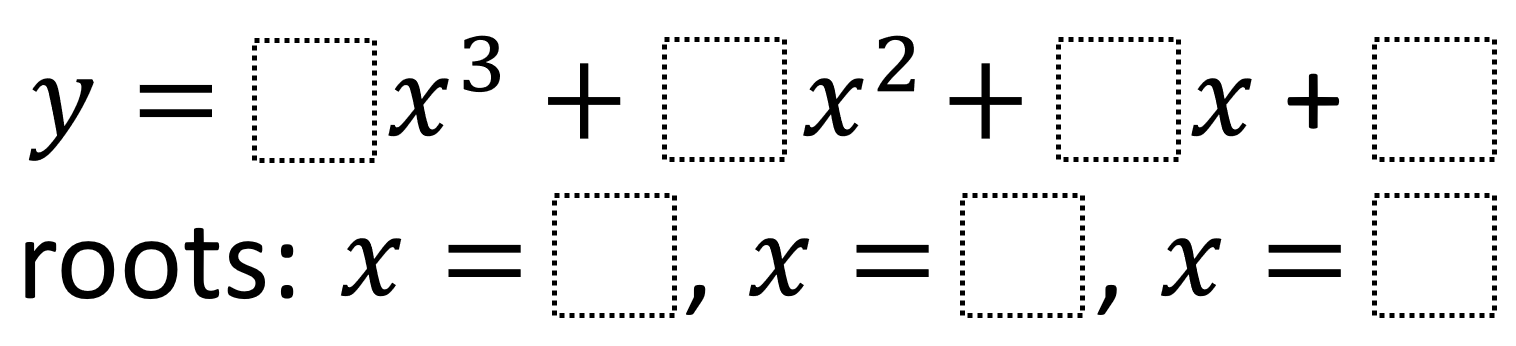# Polynomial Function Features 1

Directions: Using the integers -9 to 9, at most two times each, fill in the boxes to create a polynomial function with matching roots.### Hint

How do each of the values you can choose affect the values of the roots?

y = 1x^3 + -4x^2 + 3x + 0
roots: x = 0, x = 1, and x = 4

y = -1x^3 + -3x^2 + 4x + 0
roots: x = -4, x = 0, and x = 1

Source: Robert Kaplinsky

## Period of Trig Function 3

Directions: Using the digits 0 to 9, at most one time, fill in the blanks …